Refer to our Texas Go Math Grade 5 Answer Key Pdf to score good marks in the exams. Test yourself by practicing the problems from Texas Go Math Grade 5 Lesson 17.7 Answer Key Adjust a Budget.

Unlock the pattern

The Smith family’s net monthly income is $3,350. The graph shows the budget for the monthly expenses. The family car needs emergency repairs that cost$725. How might the family change their budget to pay for the car repairs and balance the budget?

Balance the budget.

The family income is $3,350. What is the total of the budgeted expenses? _____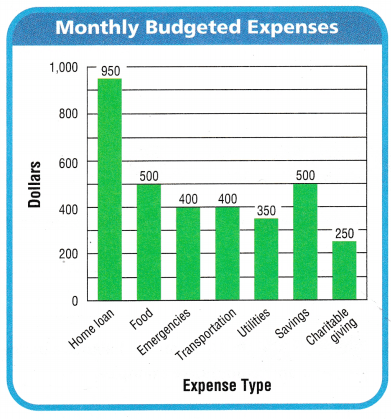How much money is budgeted for emergencies? ____ So they need ___ – ____ or ____ more to pay for the car repairs. The family could try to decrease expenses, such as ____ The family could try to increase income by _____ The family could withdraw money from their ___ this month and make it up in future months. Answer: The family income is$3,350.
The total budgeted expenses $3,350 The money budgeted for emergencies is$400.
So they need $725 –$400 or $325 more to pay for the car repairs. The family could try to decrease expenses, such as savings. The family could try to increase income by$325.
The family could withdraw money from their savings this month and make it up in future months.

Share and Show

Use the graph at the right for 1-3.

Question 1.
Ryan receives an allowance of $15 each week. He works shelving books at the library 4 times a week and receives$20 each time. He also gets $5 each week when he completes his chores at home. Is Ryan’s budget balanced? Explain. Answer:Ryan’s income$15 + 4x($20) +$5 = $100 Ryan’s expenses$10 + $20 +$12 + $10 +$28 + $20 =$100
Ryan’s budget is balanced because both income and expenses are equal.
Explanation:
In the above graph we can observe Ryan’s weekly budget. In that graph we can see Ryan’s expenses types and dollars. Add all the expenses in the graph then the sum is $100. Total expenses of Ryan’s is$100. Ryan receives an allowance of $15 each week. He works shelving books at the library 4 times a week and receives$20 each time. He also gets $5 each week when he completes his chores at home. Add all the Ryan’s income the sum is$100. Ryan’s total income is $100. Ryan’s budget is balanced because both income and expenses are equal. Question 2. Ryan does not do his chores this week. How much less is his income than his expenses? Answer: Ryan does not do his chores this week. Ryan’s income$15 + 4x($20) =$95
Ryan’s expenses
$10 +$20 + $12 +$10 + $28 +$20 = $100 Ryan’s income is$5 less than his expenses.
Explanation:
Ryan receives an allowance of $15 each week. He works shelving books at the library 4 times a week and receives$20 each time. Ryan’s income is calculated by adding all the earnings. Ryan’s total income is $95. Ryan’s expenses are calculated by using the graph Ryan’s weekly budget. Ryan’s total expenses are$100. So, Ryan’s income is $5 less than his expenses. Question 3. Explain two ways Ryan might be able to balance his budget when he does not do his chores. Answer: Ryan’s income$15 + 4x($20) =$95
First way to balance his budget when he does not do his chores.
$10 +$20 + $12 +$10 + $28 +$15 = $95 By reducing$5 on clothing expenses Ryan’s budget is balanced.
Second way to balance his budget when he does not do his chores.
$10 +$20 + $10 +$10 + $25 +$20 = $95 By reducing$2 on music and games, and $3 on books Ryan’s budget is balanced. Explanation: Ryan’s income is$95 when he does not do his chores. We can balance the budget in two ways. First way is reducing the clothing expenses by $5 then Ryan’s weekly expenses are$95. Ryan’s budget is balanced. Second way is reducing $2 on music and games, and$3 on books then Ryan’s weekly expenses are $95. Ryan’s budget is balanced. Problem Solving Question 4. Write Math How can you determine if a budget is balanced? Answer: If the total income is equal to the total expenses then we called the budget is balanced. Question 5. Write Math How can you balance a budget if the expenses are greater than income? Give examples. Answer: If the expenses are greater than income then we have to balance the budget buy reducing the expenses. Example Mia earns$15 from babysitting and $9 from dog-walking each week. Her weekly allowance is$15. Mia spent $5 on snack and$8 on music and games. She spent $10 on clothing and$20 in savings. How can Mia balance a budget if the expenses are greater than income?
Mia’s earning
$15 +$9 + $15 =$39
Mia’s expenses
$5 +$8 + $10 +$20 = $43 Here expenses are greater than income so we have balance the budget.$5 + $5 +$9 + $20 =$39
By reducing $3 on music and games, and$1 on clothing Mia’s budget is balanced.
Explanation:
In the above example the expenses are greater than income. So Mia reduced the expenses and balanced the budget.

Question 6.
Think about your weekly expenses. Name an expense that is easy to reduce and one that is not.

Problem Solving

Question 7.
Representations Michelle is a cook. Her net income is $3,200 per month. Is Michelle’s monthly budget balanced? Explain. Answer:Michelle net income is$3,200.
Michelle’s expenses is $3,559. Michelle’s expenses is greater than income. So Michelle’s monthly budget is not balanced. Explanation: Michelle is a cook. Her net income is$3,200 per month. In the above table we can observe Michelle’s expenses. Add all the expenses then the sum is $3,559. Michelle’s expenses is greater than income. So Michelle’s monthly budget is not balanced. Question 8. H.O.T. Multi-Step How can Michelle adjust the expenses in her budget to balance it? Use the column to the right to change amounts so her budget will be balanced. Answer: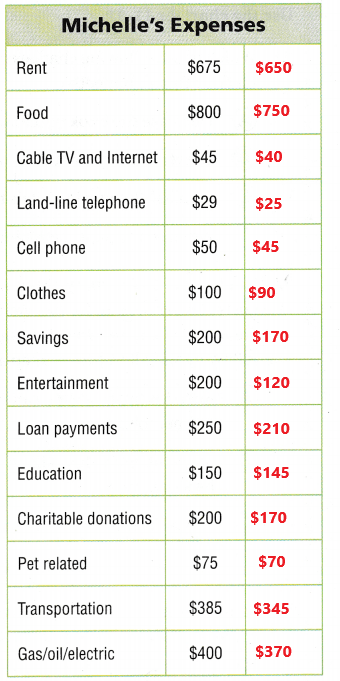Michelle’s net income is$3,200.
Michelle’s expenses is $3,200. Michelle adjusted the expenses in her budget. So Michelle’s monthly budget is balanced. Explanation: In the above table we can observe Michelle’s expenses. Michelle’s net income is$3,200. Michelle’s expenses before budget adjustment is $3,559. In the above table the column to the right is used to change amounts. The new expenses are added into the column. So that the budget is balanced. Question 9. H.O.T. Michelle decides to give up her land-line telephone and reduce her entertainment budget to$50. She also decides to cater two parties to earn $250 for each party. Use Michelle’s numbers to calculate if there is enough money left over to buy an oven for$312 Explain your reasoning.Michelle income is $3,200. Michelle’s decides to cater two parties to earn$250 for each party.
$250 x 2 =$500
Michelle’s total income
$3,200 +$500 = $3,700 Michelle’s expenses$3,559 – $150 –$29 = $3,380$3,700 – $3,380 =$320
Michelle’s have enough money left over to buy an oven for $312. Explanation: Michelle decides to give up her land-line telephone and reduce her entertainment budget to$50. Her land line telephone is $29. So subtract$150 and $29 from$3,559 then the difference is $3,380. Michelle’s expenses is$3,380. She also decides to cater two parties to earn $250 for each party. Multiply$250 with 2 then the product is $500. Michelle’s income is$3,200. Add $500 with$3,200 then the sum is $3,700. Michelle’s total income is$3,700. Subtract $3,380 from$3,700 then the difference is $320. Michelle’s have enough money left over to buy an oven for$312.

Question 10.
Multi-Step Alex works in a call center answering phones. He earns $13 per hour after taxes and works 35 hours per week. Each week, Alex is also paid$195 after taxes at his part-time job. If Alex balances his budget, how much are his weekly expenses?
1 hour = $13 35 hours =$?
$13 x 35 hours =$455
Each week, Alex is also paid $195 after taxes at his part-time job.$455 + $195 =$650
Alex’s total income is $650. If Alex balances his budget then his weekly expenses are$650.
Explanation:
Alex works in a call center answering phones. He earns $13 per hour after taxes and works 35 hours per week. Multiply$13 with 35 hours then the product is $455. Each week, Alex is also paid$195 after taxes at his part-time job. Add $455 with$195 then the sum is $650. If Alex balances his budget then his weekly expenses are$650.

Fill in the bubble completely to show your answer. Use the chart for 11-12.

Question 11.
Which of the following changes can be made to balance Dean’s budget?
(A) Decrease the book purchases to $15. (B) Decrease the paper route income to$15.
(C) Increase the movie rentals to $15. (D) Increase the paper route income to$22.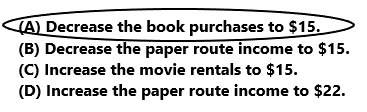Dean’s income
$25 +$20 = $45 Dean’s expenses$18 + $20 +$12 = $50 To balance Dean’s budget Dean’s decrease the book purchases to$15.
So, option A is correct.
Explanation:
In the above chart we can observe Dean’s January budget. Add all the Dean’s earning then the sum is $45. By adding all the Dean’s expenses the sum is$50. Dean’s expenses are greater than income. To balance the budget Dean’s need to decrease the book purchase to $15. So, option A is correct. Question 12. Multi-Step In February, Dean would like to get a new cell phone plan that costs$30 per month. He can increase his paper route to $25 per month. He would like to continue buying books and renting movies. Which of the following will allow him to keep a balanced budget? (A) Keep his expenses the same, and increase his income by$10 per month.
(B) Spend no more than $15 on books and increase his income by$5 per month.
(C) Spend no more than $10 on books and$10 on movies.
(D) Spend no more than $15 on books and$7 on movies.Dean like to get a new cell phone plan that costs $30 per month.$30 + $20 +$12 = $62 In February, Dean expenses is$62.
Dean increase his paper route to $25 per month.$25 + $25 =$50
In February, Dean income is $50. Dean’s February budget is not balanced because expenses is greater than income. The balanced budget is obtained by spending not more than$10 on books and $10 on movies. So Option C is correct. Explanation: In February, Dean would like to get a new cell phone plan that costs$30 per month. By adding all the expenses the sum is $62. In February, Dean expenses is$62. He can increase his paper route to $25 per month. He can continue buying books and renting movies. Add all the income the sum is$50. Dean’s February budget is not balanced because expenses is greater than income. The balanced budget is obtained by spending not more than $10 on books and$10 on movies. So Option C is correct.

Question 13.
Sandy’s expenses are greater than her income. Which of the following could Sandy increase in order to balance her budget?
(A) loan payment
(C) charitable donations
(D) hourly pay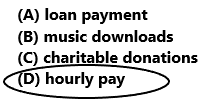Option D is correct.
Explanation:
Sandy’s expenses are greater than her income. Sandy need to increase his hourly pay in order to balance her budget. If hourly pay increases then income will increases then budget is balanced. So, option D is correct.

Texas Test Prep

Question 14.
Natalia’s net income is $28,000 per year. She would like to have a balanced budget. If her other expenses are three-fourths as much as her income, how much can she save each year? (A)$28,000
(B) $700 (C)$21,000
(D) $7,000 Answer:Natalia’s net income is$28,000 per year.
Her other expenses are three-fourths as much as her income.
(3/4) x $28,000 =$21,000
Natalia’s expenses are $21,000.$28,000 – $21,000 =$7,000
Natalia save $7,000 each year. So, option D is correct. Explanation: Natalia’s net income is$28,000 per year. Her other expenses are three-fourths as much as her income. Multiply (3/4) with $28,000 the product is$21,000. Natalia’s expenses are $21,000. Subtract expenses from income then the difference is$7,000. Natalia save $7,000 each year. So, option D is correct. ### Texas Go Math Grade 5 Lesson 17.7 Homework and Practice Answer Key Use the graph at the right for 1-2. Question 1. Jordan earns$10 each weekday for chores she completes after school. Her mother pays her $20 on Saturday and$15 on Sunday for helping with her catering business. Is Jordan’s budget balanced? Explain.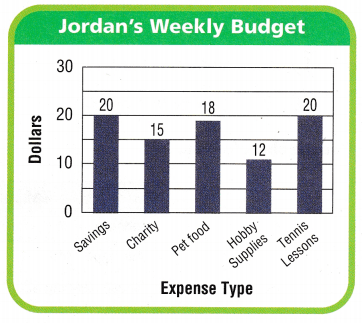Jordan’s income
$10 +$20 + $15 =$45
Jordan’s expenses
$20 +$15 + $18 +$12 + $20 =$85
Jordan budget is not balanced because expenses are greater than income.
Explanation:
Jordan earns $10 each weekday for chores she completes after school. Her mother pays her$20 on Saturday and $15 on Sunday for helping with her catering business. Add all the earnings then the sum is$45. In the above chart we can observe Jordan’s expenses. Add all the expenses then the sum is $85. Jordan budget is not balanced because expenses are greater than income. Question 2. Next week, Jordan has a tennis match on Saturday and cannot help her mother. What would be the difference between her income that week and her expenses? Answer: Jordan’s income$10 + $15 =$25
Jordan’s expenses
$20 +$15 + $18 +$12 + $20 =$85
$85 –$25 = $65 The difference between Jordan income and her expenses are$65.
Explanation:
Jordan earns $10 each weekday for chores she completes after school. Jordan has a tennis match on Saturday and cannot help her mother. Her mother pays her$15 on Sunday for helping with her catering business. Add all the income then the sum is $25. Jordan’s expenses are$85. Subtract income from expenses then the difference is equal to $65. The difference between Jordan income and her expenses are$65.

Problem Solving

Use the table for 3-4.

Question 3.
Jesse earns $2,500 per month after taxes, working at a car repair shop. Is his budget balanced? Explain. Answer:Jesse’s income per month is$2,500.
Jesse expenses
$795 +$750 + $80 +$225 + $125 +$200 + $200 +$150 + $55 +$100 = $2,680 Jesse’s budget is not balanced because expenses are greater than income. Explanation: Jesse earns$2,500 per month after taxes, working at a car repair shop. In the above table we can observe Jesse’s monthly expenses. Add all the expenses then the sum is $2,680. Jesse’s budget is not balanced because expenses are greater than income. Question 4. Sometimes Jesse works on his friends’ cars on the weekend and earns an extra$100 per car. How can Jesse balance his monthly budget?
Jesse’s income per month
$2,500 +$100 = $2,600 Jesse’s expenses is$2,680.
Jesse is balancing budget by decreasing some expenses.
$795 +$740 + $80 +$215 + $115 +$200 + $190 +$140 + $55 +$70 = $2,600 Jesse balanced his monthly budget by decreasing some expenses. Explanation: Sometimes Jesse works on his friends’ cars on the weekend and earns an extra$100 per car. Add net income $2,500 with$100 the sum is $2,600. Jesse’s income per month is$2,600. Jesse’s expenses is $2,680. After balancing budget by decreasing some expenses the total expenses are$2,600. Jesse balanced his monthly budget by decreasing some expenses.

Lesson Check

Question 5.
Mr. Porter wants to spend an extra $150 this weekend on a family outing to the state fair. Which of the following could be a way to adjust his budget to allow for the extra expense? (A) Work fewer hours this week. (B) Work overtime (C) Give money to charity. (D) Increase gasoline usage. Answer:Option A is correct. Explanation: Mr. Porter wants to spend an extra$150 this weekend on a family outing to the state fair. He has to adjust his budget to allow for the extra expense are that he should work fewer hours this week. So, option A is correct.

Question 6.
Alexis earns $50 a day for five days this week. Her weekly expenses are$280. How can she balance her budget?
(A) Increase her income $5 each day. (B) Increase her income$20 this week.
(C) Decrease her expenses by $30. (D) Decrease her expenses by$20.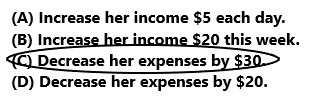Alexis income
$50 x 5 =$250
Her weekly expenses are $280.$280 – $250 =$30
To balance the budget Alexis has to decrease her expenses by $30. So, option C is correct. Explanation: Alexis earns$50 a day for five days this week. Multiply $50 with 5 then the product is$250. Her weekly expenses are $280. Subtract$250 from $280 then the difference is$30. To balance the budget Alexis has to decrease her expenses by $30. So , option C is correct. Use the chart for 7-8.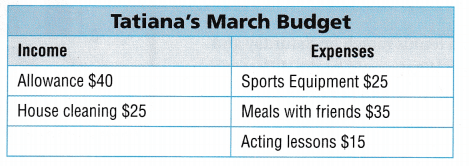Question 7. Which of the following changes can be made to balance Tatiana’s budget? (A) Decrease spending on sports equipment to$20.
(B) Increase income from house cleaning to $30. (C) Increase spending on acting lessons to$25.
(D) Decrease spending on meals with friends to $25. Answer: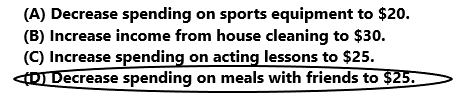Tatiana’s income$40 + $25 =$65
Tatiana’s expenses
$25 +$35 + $15 =$75
To balance Tatiana’s budget. Tatiana’s has to decrease spending on meals with friends to $25. So, option D is correct. Explanation: In the above chart we can observe Tatiana’s income and expenses. Tatiana’s income is calculated by adding all the earnings then the sum is$65. Add all the expenses then the sum is $75. To balance Tatiana’s budget. Tatiana’s has to decrease spending on meals with friends to$25. So, option D is correct.
Question 8.
Multi-Step Tatiana would like to buy soccer shoes that are on sale for $30. She will use her sports equipment budget for part of the cost. Which of the following plans will allow Tatiana to have a balanced budget? (A) increase her income by$15.
(B) Spend only $15 on meals. (C) Spend only$20 on meals and increase her house cleaning income by $15. (D) Increase her income by$60.Tatiana’s income
$40 +$25 = $65 Tatiana’s expenses$25 + $35 +$15 = $75$75 + $5 =$80
To balance Tatiana’s budget. Tatiana’s has to increase her income by $15. So, option A is correct. Explanation: In the above chart we can observe Tatiana’s income and expenses. Tatiana’s income is calculated by adding all the earnings then the sum is$65. Add all the expenses then the sum is $75. Tatiana would like to buy soccer shoes that are on sale for$30. She will use her sports equipment budget for part of the cost. The expenses are $80. To balance the budget Tatian’s need to increase her income by$15. So, option A is correct.

Question 9.
Multi-Step Duke works at a grocery store sacking groceries. He earns $12 per hour after taxes and works 24 hours each week. His weekly expenses total$324. How many more hours would Duke need to work to balance his budget?
(A) 1 hour
(B) 2 hours
(C) 4 hours
(D) 3 hours1 hour = $12 24 hours = ?$12 x 24 = $288 Duke income is$288
His weekly expenses total $324.$324 – $288 =$36
$12 x 3 hours =$36
Duke works at a grocery store sacking groceries. He earns $12 per hour after taxes and works 24 hours each week. Multiply$12 with 24 hours the product is $288. His weekly expenses total$324. Subtract $288 from$324 the difference is \$36. Duke need to work 3 hours to balance his budget. So, option D is correct.